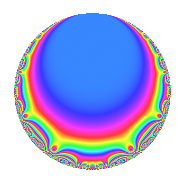# Properties

 Label 600.2.b.aLevel 600 Weight 2 Character orbit 600.b Analytic conductor 4.791 Analytic rank 0 Dimension 2 CM disc. -8 Inner twists 4

# Learn more about

## Newspace parameters

 Level: $$N$$ = $$600 = 2^{3} \cdot 3 \cdot 5^{2}$$ Weight: $$k$$ = $$2$$ Character orbit: $$[\chi]$$ = 600.b (of order $$2$$ and degree $$1$$)

## Newform invariants

 Self dual: No Analytic conductor: $$4.79102412128$$ Analytic rank: $$0$$ Dimension: $$2$$ Coefficient field: $$\Q(\sqrt{-2})$$ Coefficient ring: $$\Z[a_1, a_2]$$ Coefficient ring index: $$1$$ Sato-Tate group: $\mathrm{U}(1)[D_{2}]$

## $q$-expansion

Coefficients of the $$q$$-expansion are expressed in terms of $$\beta = \sqrt{-2}$$. We also show the integral $$q$$-expansion of the trace form.

 $$f(q)$$ $$=$$ $$q$$ $$+ \beta q^{2}$$ $$+ ( 1 - \beta ) q^{3}$$ $$-2 q^{4}$$ $$+ ( 2 + \beta ) q^{6}$$ $$-2 \beta q^{8}$$ $$+ ( -1 - 2 \beta ) q^{9}$$ $$+O(q^{10})$$ $$q$$ $$+ \beta q^{2}$$ $$+ ( 1 - \beta ) q^{3}$$ $$-2 q^{4}$$ $$+ ( 2 + \beta ) q^{6}$$ $$-2 \beta q^{8}$$ $$+ ( -1 - 2 \beta ) q^{9}$$ $$-2 \beta q^{11}$$ $$+ ( -2 + 2 \beta ) q^{12}$$ $$+ 4 q^{16}$$ $$-4 \beta q^{17}$$ $$+ ( 4 - \beta ) q^{18}$$ $$+ 2 q^{19}$$ $$+ 4 q^{22}$$ $$+ ( -4 - 2 \beta ) q^{24}$$ $$+ ( -5 - \beta ) q^{27}$$ $$+ 4 \beta q^{32}$$ $$+ ( -4 - 2 \beta ) q^{33}$$ $$+ 8 q^{34}$$ $$+ ( 2 + 4 \beta ) q^{36}$$ $$+ 2 \beta q^{38}$$ $$-8 \beta q^{41}$$ $$+ 10 q^{43}$$ $$+ 4 \beta q^{44}$$ $$+ ( 4 - 4 \beta ) q^{48}$$ $$+ 7 q^{49}$$ $$+ ( -8 - 4 \beta ) q^{51}$$ $$+ ( 2 - 5 \beta ) q^{54}$$ $$+ ( 2 - 2 \beta ) q^{57}$$ $$+ 10 \beta q^{59}$$ $$-8 q^{64}$$ $$+ ( 4 - 4 \beta ) q^{66}$$ $$-14 q^{67}$$ $$+ 8 \beta q^{68}$$ $$+ ( -8 + 2 \beta ) q^{72}$$ $$-2 q^{73}$$ $$-4 q^{76}$$ $$+ ( -7 + 4 \beta ) q^{81}$$ $$+ 16 q^{82}$$ $$+ 2 \beta q^{83}$$ $$+ 10 \beta q^{86}$$ $$-8 q^{88}$$ $$+ 4 \beta q^{89}$$ $$+ ( 8 + 4 \beta ) q^{96}$$ $$+ 10 q^{97}$$ $$+ 7 \beta q^{98}$$ $$+ ( -8 + 2 \beta ) q^{99}$$ $$+O(q^{100})$$ $$\operatorname{Tr}(f)(q)$$ $$=$$ $$2q$$ $$\mathstrut +\mathstrut 2q^{3}$$ $$\mathstrut -\mathstrut 4q^{4}$$ $$\mathstrut +\mathstrut 4q^{6}$$ $$\mathstrut -\mathstrut 2q^{9}$$ $$\mathstrut +\mathstrut O(q^{10})$$ $$2q$$ $$\mathstrut +\mathstrut 2q^{3}$$ $$\mathstrut -\mathstrut 4q^{4}$$ $$\mathstrut +\mathstrut 4q^{6}$$ $$\mathstrut -\mathstrut 2q^{9}$$ $$\mathstrut -\mathstrut 4q^{12}$$ $$\mathstrut +\mathstrut 8q^{16}$$ $$\mathstrut +\mathstrut 8q^{18}$$ $$\mathstrut +\mathstrut 4q^{19}$$ $$\mathstrut +\mathstrut 8q^{22}$$ $$\mathstrut -\mathstrut 8q^{24}$$ $$\mathstrut -\mathstrut 10q^{27}$$ $$\mathstrut -\mathstrut 8q^{33}$$ $$\mathstrut +\mathstrut 16q^{34}$$ $$\mathstrut +\mathstrut 4q^{36}$$ $$\mathstrut +\mathstrut 20q^{43}$$ $$\mathstrut +\mathstrut 8q^{48}$$ $$\mathstrut +\mathstrut 14q^{49}$$ $$\mathstrut -\mathstrut 16q^{51}$$ $$\mathstrut +\mathstrut 4q^{54}$$ $$\mathstrut +\mathstrut 4q^{57}$$ $$\mathstrut -\mathstrut 16q^{64}$$ $$\mathstrut +\mathstrut 8q^{66}$$ $$\mathstrut -\mathstrut 28q^{67}$$ $$\mathstrut -\mathstrut 16q^{72}$$ $$\mathstrut -\mathstrut 4q^{73}$$ $$\mathstrut -\mathstrut 8q^{76}$$ $$\mathstrut -\mathstrut 14q^{81}$$ $$\mathstrut +\mathstrut 32q^{82}$$ $$\mathstrut -\mathstrut 16q^{88}$$ $$\mathstrut +\mathstrut 16q^{96}$$ $$\mathstrut +\mathstrut 20q^{97}$$ $$\mathstrut -\mathstrut 16q^{99}$$ $$\mathstrut +\mathstrut O(q^{100})$$

## Character Values

We give the values of $$\chi$$ on generators for $$\left(\mathbb{Z}/600\mathbb{Z}\right)^\times$$.

 $$n$$ $$151$$ $$301$$ $$401$$ $$577$$ $$\chi(n)$$ $$-1$$ $$-1$$ $$-1$$ $$1$$

## Embeddings

For each embedding $$\iota_m$$ of the coefficient field, the values $$\iota_m(a_n)$$ are shown below.

For more information on an embedded modular form you can click on its label.

Label $$\iota_m(\nu)$$ $$a_{2}$$ $$a_{3}$$ $$a_{4}$$ $$a_{5}$$ $$a_{6}$$ $$a_{7}$$ $$a_{8}$$ $$a_{9}$$ $$a_{10}$$
251.1
 − 1.41421i 1.41421i
1.41421i 1.00000 + 1.41421i −2.00000 0 2.00000 1.41421i 0 2.82843i −1.00000 + 2.82843i 0
251.2 1.41421i 1.00000 1.41421i −2.00000 0 2.00000 + 1.41421i 0 2.82843i −1.00000 2.82843i 0
 $$n$$: e.g. 2-40 or 990-1000 Significant digits: Format: Complex embeddings Normalized embeddings Satake parameters Satake angles

## Inner twists

Char. orbit Parity Mult. Self Twist Proved
1.a Even 1 trivial yes
8.d Odd 1 CM by $$\Q(\sqrt{-2})$$ yes
3.b Odd 1 yes
24.f Even 1 yes

## Hecke kernels

This newform can be constructed as the intersection of the kernels of the following linear operators acting on $$S_{2}^{\mathrm{new}}(600, [\chi])$$:

 $$T_{7}$$ $$T_{11}^{2}$$ $$\mathstrut +\mathstrut 8$$ $$T_{23}$$ $$T_{43}$$ $$\mathstrut -\mathstrut 10$$